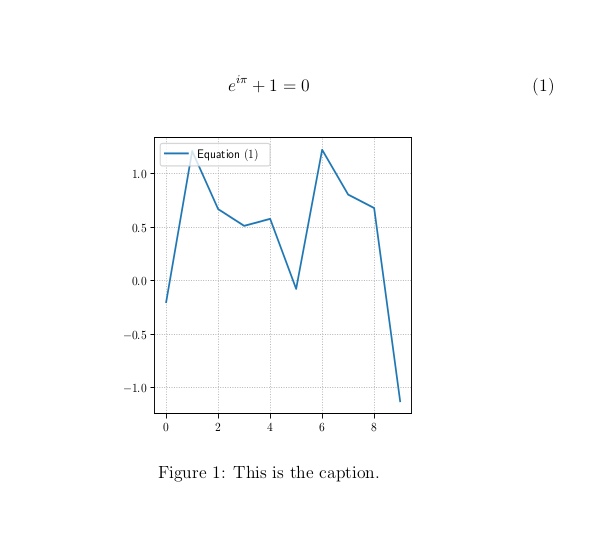Recently, I wanted to reference an equation in a LaTeX document from a figure created using matplotlib. Luckily, matplotlib has a PGF backend that makes this possible. Here’s an example:

main.tex:

So we have an labeled equation, and we’d like to create the file figure.pgf so that the equation can be referenced in the figure.

make_figure.py:

I’ve borrowed some of the settings from the matplotlib pgf page. You can apparently get quite deep into the pgf settings in matplotlib (see here for example).

Now, finally run,

That not a typo — you must run pdflatex twice to get the references correct. Here’s the output:Further, if you add \usepackage{hyperref} to main.tex, the equation reference in the figure is hyperlinked to the equation itself!